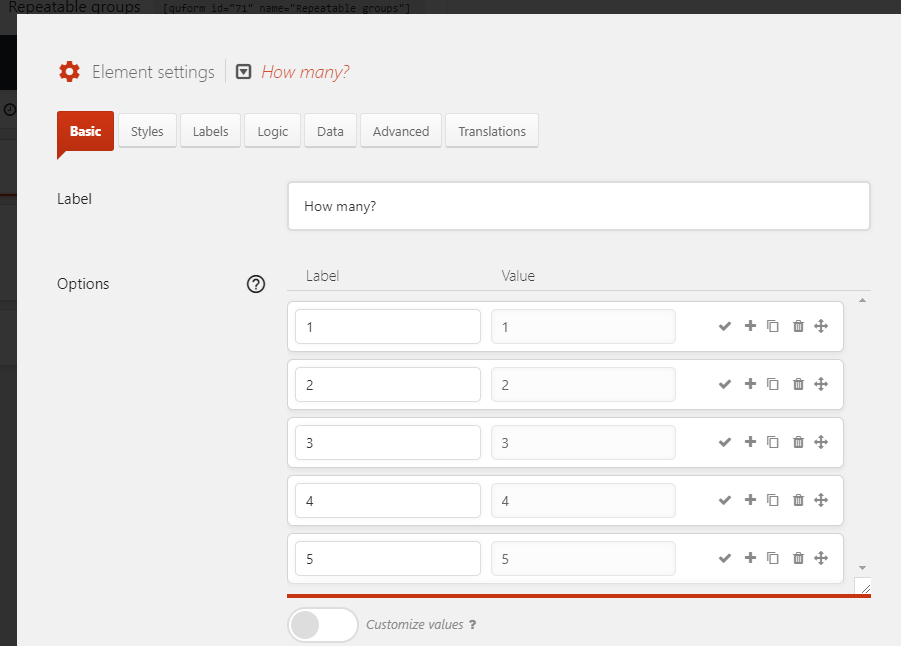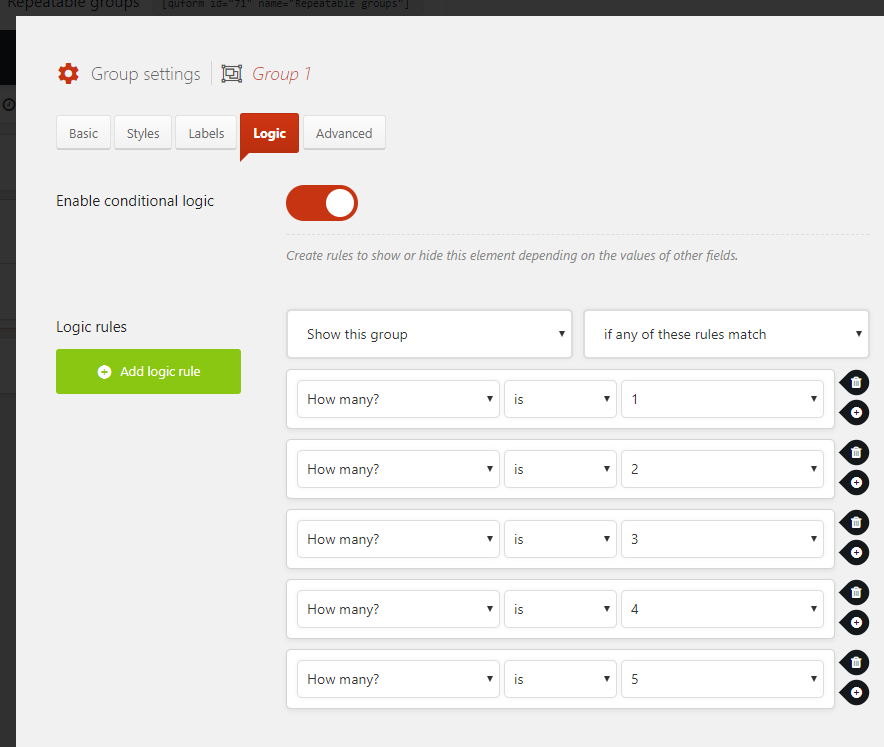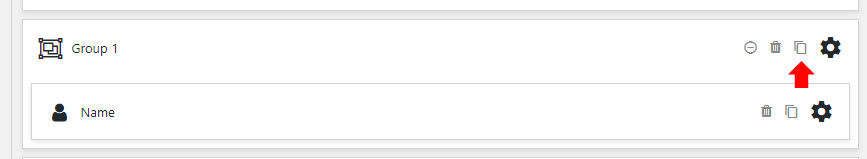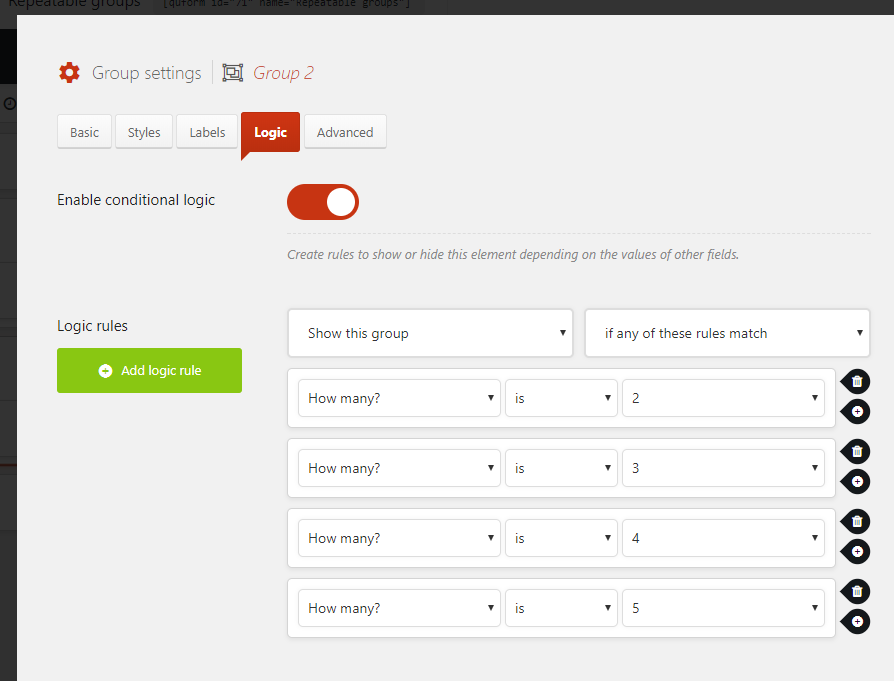# Repeatable groups

While it’s not yet possible to infinitely repeat fields or a group of fields on the front end of the form, you can create a number of groups or elements in the form builder and use a Select Menu with conditional logic to show them. In this example we will set up a form that will allow the user to show 1 to 5 groups.

## Step 1

Add a Select Menu element to the form. In the settings add the numbers as options for the number of groups or elements you want to support, this field will be a question to the user along the lines of “How many?”.## Step 2

Add the Group or other element that you want to be repeatable, and configure all of the elements inside it. We will be duplicating this so it’s best if you make it perfect now so that you don’t need to edit all of the duplicated elements later.

### Step 3

In the settings for the First group, go to the Logic tab and click Enable conditional logic, then set the logic as follows.Now duplicate the first group.Then go to the Logic tab for the duplicated (Second) group, and remove the first logic logic rule.Continue duplicating the last group and removing the first logic rule each time. The full logic for all groups is shown below.

```(Show this group) (if any of these rules match)
How many? is 1
How many? is 2
How many? is 3
How many? is 4
How many? is 5

(Show this group) (if any of these rules match)
How many? is 2
How many? is 3
How many? is 4
How many? is 5

(Show this group) (if any of these rules match)
How many? is 3
How many? is 4
How many? is 5

(Show this group) (if any of these rules match)
How many? is 4
How many? is 5

(Show this group) (if any of these rules match)
How many? is 5```

To support numbers greater than 5 you will need more logic rules, but the process is the same – duplicate the last group and remove the first logic rule each time.

Be inspired. © 2020 ThemeCatcher Ltd. 20-22 Wenlock Road, London, England, N1 7GU | Company No. 08120384 | Built with React | Privacy Policy